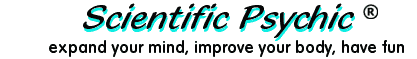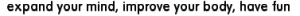IndexThe Leaning Tower Problem - part 2

It is said that Galileo (1564-1642) dropped balls of various weights from the top of the Leaning Tower of Pisa to refute an Aristotelian belief that heavier objects fall faster than lighter objects.

If the balls were dropped from a height of 54 meters, how fast were the balls going when they hit the ground?

The speed of an object under constant acceleration is given by the formula:

speed = acceleration × time + intial speed

We know from part 1 of the problem that the balls take 3.3 seconds to reach the ground. Substituting initial speed = 0, time = 3.3 seconds and acceleration = 9.8 meters/second2, we get:

speed = 9.8 meters/second2 × 3.3 seconds

speed = 32.3 meters/second (116 Kilometers/hour)One reader submitted the following comment:

If the answer should be given mathematically, (i.e., The speed of the balls worked out from the height, etc.), then the question should ask what speed the balls were going BEFORE impact. Because it asks what speed the balls were going when they DID hit the ground, the answer is zero. Any object hitting the ground immediately has its velocity reset to zero, i.e., it stops.